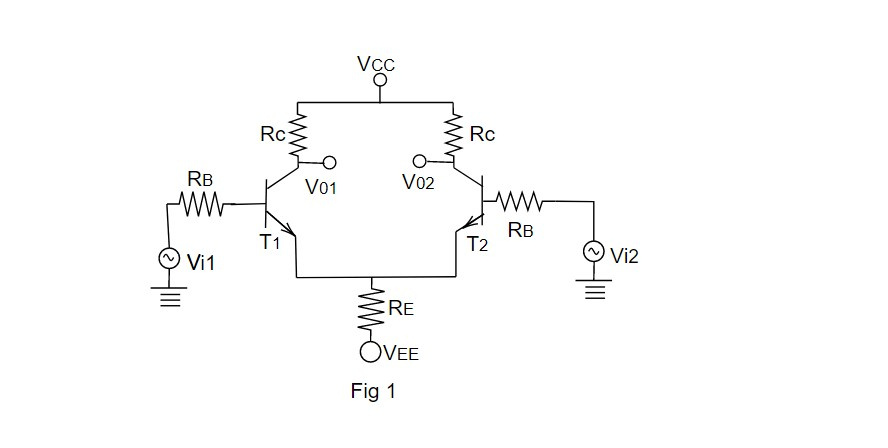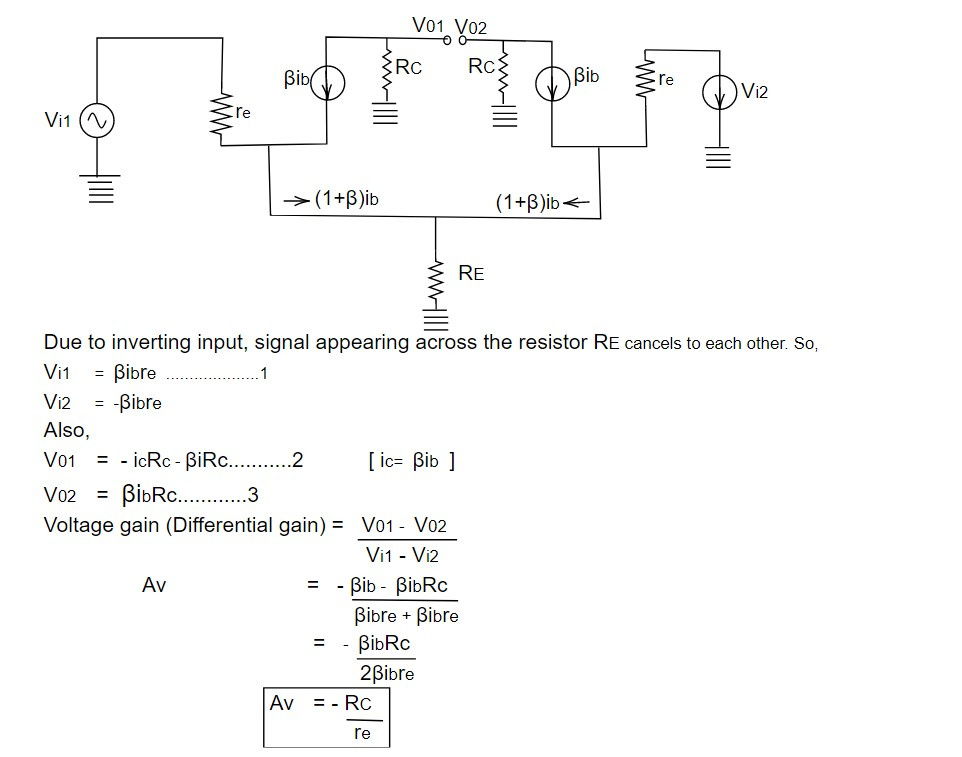# Differential Amplifier

Differential Amplifier is a very important and basic circuit of IC devices. It has two transistors T1 and T2 are assumed to be identical. The transistor are used in CE configuration as shown in figure.The signals are given to each on both of these bases and outputs are taken from each or both of the capacitors of the identical transistors T1 and T2 as shown in figure. It is widely used as integrated circuit.

If an input signal is applied to either input and other input connected to the ground, the operation is referred to as a single ended.

If two polarity input signals are applied, the operation is referred to as a Double ended.

If the same input is applied to both input, the operation is called 'common mode'.

Double ended differential amplifier (Differential mode)

In this amplifier, the inverted signals are applied to the inputs. Due to this, inverting signals appearing across the emitter cancels each other so that it becomes regenerating mode.

The circuit diagram of double ended (Differential mode) is shown in figure above.

AC equivalent circuit of fig 1